Next Article in Journal / Special Issue
Use of McKibben Muscle in a Haptic Interface
Previous Article in Journal / Special Issue
A Comparison of Robot Wrist Implementations for the iCub Humanoid
Open AccessArticle

# Development of a Novel SMA-Driven Compliant Rotary Actuator Based on a Double Helical Structure†

byRasheed Kittinanthapanya *,Yusuke Sugahara *,Daisuke MatsuuraandYukio TakedaSchool of Engineering, Tokyo Institute of Technology, Tokyo 152-8552, Japan
*
Authors to whom correspondence should be addressed.
This paper is an extended version of our paper published in Kittinanthapanya, R.; Sugahara, Y.; Matsuura, D.; Takeda, Y. A Novel SMA Driven Compliant Rotary Actuator Based on Double Helical Structure. In Proceedings of the IFToMM Symposium on Mechanism Design for Robotics, Udine, Italy, 11–13 August 2018.
Robotics 2019, 8(1), 12; https://doi.org/10.3390/robotics8010012
Received: 15 January 2019 / Revised: 11 February 2019 / Accepted: 13 February 2019 / Published: 18 February 2019

## Abstract

This paper proposes a new shape memory alloy (SMA)-driven compliant rotary actuator that can perform both passive and self-actuated motions. This SMA actuator is suitable as a redundant actuation part in a parallel robot joint to assist with singularity postures where the robot might lose the ability to maintain the position and orientation of the end effector. The double helical compliant joint (DHCJ) was chosen as a candidate mechanism; it can act in soft compliance with linear characteristics and a wide range of motion. The experimental results validated that the proposed model can be used to simulate the DHCJ behavior. The use of this mechanism exhibits advantages such as one-axis rotational motion, linear behavior even for a compliant mechanism, stiffness in the other axes of motion, and compact size. SMA leaves (strips) were used as actuation parts, and a single SMA leaf was tested before combining with the double helical frame as an SMA actuator. The prototype was fabricated, and necessary parameters such as deflection angle, temperature, torque, and stress–strain were collected to define the model for a controller. This actuator is controlled by a feedforward controller and provides rotational motion for both forward and reverse sides with a maximal range of 40 degrees.
Keywords:

## 1. Introduction

Shape Memory Alloy (SMA) is a special material that can recover itself to its original shape when the proper heat is applied. In the robotics field, SMA has been used as a smart actuator by providing electrical current directly to its part. Generally, the SMA part works together with a bias spring  because after SMA is energized, it cannot return to its initial state, therefore a bias spring would be able to bring SMA back to its initial state. Using SMA material as a smart actuator, it shows advantages such as high force per weight ratio, simple electrical current drive, and operation in silence . The SMA actuator is better to apply in miniature applications than massive machines and the operation frequency is quite low compared to the other conventional actuators. SMA’s actuation can be designed as translational and angular motion. Normally, the common shapes of SMA, which are produced easily, are wire-shaped, spring-shaped and strip-shaped. Farias  proposed a four-fingered robot hand by using SMA wire as an actuation part. Hamid  also designed the morphing wing mechanism by using an SMA wire and a bias spring. Yuan  designed the compliant actuator with SMA wire inside a plastic structure. These SMA actuators were designed for a specific applications. Wire and spring types are the most practical type for almost of SMA researches. For wire and spring types, the memorized shape is often set as a straight shape and contraction shape which both provide translational motion. If an angular or rotational motion is desired, an external mechanism is required to transform SMA movement into desired motion. Raynaerts and Van Brussel  proposed a technique and design aspect of shape memory alloy actuators such as a cooling system design, but this will cause the structure to become vast and massive.
To maintain the stiffness of the actuator, avoid unnecessary weight, and generalize it in a manner similar to a motor, the special compliant mechanism is considered as a frame for the actuator instead of a normal spring or other mechanism. In this study, the bias spring was replaced with a compliant mechanism called a double helical compliant joint (DHCJ) . A similar concept was used with another smart material; Modler  designed a compliant mechanism integrated with a piezo ceramic actuator for a flap mechanism, and Hoang and Chen  designed a flexure parallel mechanism with the concept of selective actuation. The DHCJ consists of two materials: a hard material for the frame and a flexible material for the deformation part. This combination provides the actuator with sufficient stiffness and rotational motion. In order to move this joint, one frame is fixed to the ground and the other is pushed or pulled as a passive joint. The motion of this joint does not require any bearings or contacting parts, so this compliant joint can provide high-precision motion without lubricants, and can be washable. The purpose of this paper is to propose a new SMA compliant actuator based on the double helical structure. The characteristics of this SMA actuator were experimentally investigated, and the related parameters were determined by the experimental apparatus.

## 2. Design of the Double Helical Compliant Joint

#### 2.1. Design Concept of the DHCJ

The DHCJ was originally designed by Yonemoto et al.  for a large-workspace compliant mechanism by using soft material for the deformation parts and rigid material for the frame. This compliant joint is a passive joint, which is suitable for application as a parallel manipulator. It consists of two similar frames with the same coordinate system, but each frame is placed 180 degrees in the z-axis from the other as shown in Figure 1a,b and connected together by leaf springs as shown in Figure 1c,d. The original DHCJ was designed to use 16 leaf springs with the overall size of $40 × 40 × 100$ mm. As shown in Figure 2a, one frame is removed to show that the leaf springs are placed symmetrically at the mirror plane. There are eight pieces at the front side and eight pieces at the back side as shown in Figure 2b. This kind of arrangement can constrain undesired motion from the other five axes so that the joint rotates only around the z-axis. Accordingly, the joint can be estimated as working as a one degree-of-freedom mechanism .

#### 2.2. Modeling of the DHCJ

In order to model the DHCJ, the chained-beam constraint model (CBCM) was used because it is simple to use in calculation and simulation of the result. The CBCM is a numerical method which developed by Ma and Chen  to be used in a compliant mechanism with various constraint conditions. The DHCJ can be considered as 16 elastic beams fixed at one end and constrained at the other end to move in a circular path. The same load was applied to each elastic beam, so only just one model multiplied by 16 would yield the whole stiffness of the mechanism, as shown in Figure 3. The experiment were performed to verify that the proposed method can predict the actual behavior of the DHCJ. The apparatus is shown in Figure 4, where the DHCJ is driven from an initial angle to the maximum angle. The results from Figure 5 show that the DHCJ acts as a soft linear compliant mechanism, which is suitable for adoption with other mechanisms as a rotational motion component. From the characteristics graphs, the double helical structure with proper arrangement of the leaf springs can transform the nonlinear system mechanism (large deflection of an elastic beam) to become simply a spring-like mechanism.

## 3. Design of the SMA Actuator

This section describes the design of the SMA component and the DHCJ in combination as a special compliant SMA actuator. The idea is to keep the size compact, by replacing some leaf springs of the DHCJ with SMA, and without increasing the external mass of the system. When no power is applied to the system, the actuator behaves as a passive compliant joint. When the proper energy in applied to it, the actuator transforms from a passive to an active component. The modeling and the idea of this conceptual design are shown.

#### 3.1. Modeling of the SMA Leaf

SMA is a special material whose phase changes when the proper heat is applied to it. At the low-temperature or martensite phase (M), the crystalline structure of an SMA is quite flexible and can be easily deformed into new shapes when force is applied. Upon heating, the crystalline structure returns to a memorized configuration in austenite phase (A). The transformation of A to M and M to A are characterized by four temperatures: $A s$ and $A f$ are the start and finish temperatures, respectively, for the A phase; $M s$ and $M f$ are the start and finish temperature, respectively, for the M phase. The phase transformation curve can be expressed in terms of a martensite fraction, $ξ$. The martensite fraction is bounded between 0 (fully austenite) and 1 (fully martensite) as shown in Figure 6. As seen in the phase transformation, SMAs show significant hysteresis during the two transformations. Many studies show that the behavior of SMA depends not only on temperature, but also on the stress acting on it. At any value of stress or temperature, the material can be in one of three states: fully martensite, fully austenite, or a mixture of austenite and martensite. The phase transformation of the SMA can be expressed as
$ξ M → A = 0.5 [ c o s ( a A ( T − A s ) − a A C A σ ) + 1 ] ξ A → M = 0.5 [ c o s ( a M ( T − M f ) − a M C M σ ) + 1 ] ,$
where $a A = π / ( A f − A s )$ and $a M = π / ( M s − M f )$, are material constants. T is the applied temperature, $σ$ is the pre-stress, and $C A$ and $C M$ are the slope of the stress and transformation temperature from experimental data.
For simplicity, for the first prototype, the one-dimensional constitutive model from Liang and Roger  was used to describe the SMA behavior as shown in Equation (2).
$σ − σ 0 = E ( ϵ − ϵ 0 ) + Ω ( ξ − ξ 0 ) ,$
where $σ$ and $σ 0$ represent the stress and the pre-stress of the SMA actuator; E is the Young’s modulus and $ϵ$ and $ϵ 0$ are the current and initial strain; $ξ$ and $ξ 0$ are the current and initial value of martensite fraction and $Ω$ is the transformation coefficient of the material constant. The coefficient can be obtained from the residual strain and modulus of elasticity as $Ω =$$− E$$ϵ L$. From this equation, when SMA changes its phase from martensite to austenite, the strain changes because of the SMA motion. The change from the right-hand side of the equation causes the SMA to generate stress which turn induces force and torque to the system. In this research, the SMA strip type was designed in a similar shape as the leaf spring in DHCJ as shown in Figure 7. The memorized shape (austenite phase shape) was set as a U-shape as shown in Figure 8. The material composition of the SMA using in this research is NiTi-Cu with $49.2 %$ of Ni, $5.8 %$ of Cu and the remaining of Ti. Considering a single leaf, with one end was fixed and the other end was bent in a circular path, the forward kinematics transforms the deflection angle $θ$ to strain $ϵ$ by considering the arc shape as shown in Figure 9. When the SMA leaf bends into each angle, the deflection angle $θ$ is equal to arc angle $ψ$, so the strain can be expressed in term of deflection angle as
$ϵ = − ψ ( r − t 2 ) − l l ,$
where t is the leaf’s thickness, l is the leaf’s length, $ψ$ is the arc angle (where $ψ = θ$) and r is the radius of curvature, where $r = ( l / 2 ) / t a n ( ψ / 2 )$.
In the robotics field, the proper way to energize the SMA is to use electrical power, Ramio and Edwin  proposed a classical differential equation that expresses the heat store in SMA as
$ρ C V d T d t = i 2 R − h A ( T − T e )$
where $ρ$ is the density of the SMA, C is the specific heat, V is the volume of the SMA, i is the electrical current, R is the elements electrical resistance, h is the heat-exchange coefficient between the SMA and the surrounding air, A is the surface area of the SMA, $T e$ is the environmental temperature and T is the SMA temperature at time t.
When electrical current is applied to the SMA leaf, it can generate a bending moment, which can be considered as generated torque $τ g$ from the SMA. The equation of rotational motion can be expressed as
$τ g = J θ ¨ + D θ ˙ + k θ ,$
where J is the moment of inertia, D is the damping coefficient, k is the rotational stiffness and $θ$ is the deflection angle.
By combining each elements as proposed previously, the overall block diagram of the SMA driven compliant actuator is as shown in Figure 10. First, the proper voltage was applied to the SMA strip; then from the heat transfer model of Equation (4), the heat changes the crystalline structure of the SMA from martensite (initial shape) to austenite state (memorized shape) as expressed in Equation (1). Those changes induced the SMA to generate stress or torque on the system as expressed in Equation (2). Because of the input torque, the mechanical system generated motion according to the dynamic parameters and yielded angular motion as the output. From this concept block diagram, the feed-forward controller is considered to evaluate the precision of the model from theory to practice.

#### 3.2. Characteristics of a Single SMA Leaf

In order to understand the physical behavior of the SMA before combining it with the DHCJ frame, a single SMA leaf was tested to validate the model. A driver circuit and proper mechanical structure were also tested to validate the concept. The approximated kinematics of DHCJ with the SMA leaf and leaf spring are shown in Figure 11. According to the DHCJ motion that can rotate in a circular path, the rigid link that acts as the radius arm is attached at the upper and lower parts. Two bearings are used to constrain the motion only around the principal axis. There are two SMA leaves for forward and reverse driving. A pre-bent angle was added to both leaves to avoid the beam buckling shape problem. When the SMA is energized at the forward side, the reverse SMA is bent to a straight shaped. Similarly, when the reverse SMA is energized, the forward SMA will become straight either as shown in Figure 12.
A pulse-width modulation (PWM) drive was used because it is an efficient way to control the SMA and it exhibits a linear proportional relationship between the power and duty cycle. To provide the proper amount of electrical energy, a high drain lithium ion battery (IMR 18650) was used. To generate heat in a short time, the SMA strip was shorted-circuited. For this experiment, the circuit shown in Figure 13 was used as the SMA driver. The various pre-bent angles of SMA as shown in Figure 14 were tested with the same configuration, and the result shows that from no pre-bent angle to a small pre-bent angle, the SMA seemed to give much power to drive, but in fact the opposite SMA resisted the motion, resulting in only a small angle movement. A large pre-bent angle, such as 45 degrees, yielded the expected result in which the overall motion turned by only a small angle because the initial point was already half of the memorized shape, so the power was not enough to induce a large deflection angle. The pre-bent angle of 30 degrees was chosen because it can have the largest deflection angle at 30 degrees for each side.
The ambient temperature during testing was 20–22 $∘$C. The SMA started to rotate when it reached the temperature of 38–40 $∘$C, and reached the maximum angle of 30 degrees at a temperature of approximately 50–55 $∘$C. When the power was released, the temperature decreased to approximately 33 $∘$C while maintaining the position at the same angle; then the bias spring tried to rotate the SMA back to its initial position. The deflection angle and temperature of the SMA are represented with respect to time by changing the duty cycle to $45 %$, $60 %$ and $75 %$ as shown in Figure 15. A 45% duty cycle yielded a lower temperature, but it required more time to reach the maximum angle. Figure 15a shows that the higher voltage can decrease the SMA response time, but it also caused higher temperature. When the energy was released during the “off” time, all of the SMA’s temperature dropped down similarly from the maximum temperature to approximately 30 $∘$C, then linearly decreased to room temperature as shown Figure 15b. The testing results show that the SMA strip works from 45% to 75% duty cycle with 4 V amplitude and 10 A drawing currents; for lower duty cycles, the SMA only slightly changed and never reached the maximum angle of 30 $∘$C.

#### 3.3. An Actuator Design

The information from the test described previously was used to design the combination of SMA strip and double helical structure. The SMA leaves were divided into four pieces for the forward SMA and four pieces for the reverse SMA, and all of the SMA components were pre-bent to some angle. The leaf springs were chosen as eight pieces, with a straight shape and half of the width in size to make the actuator soft in the z-axis rotation and stiff in the other axes, as shown in Figure 16a,b. The special double helical frame for the SMA actuator was designed according to the requirement of the SMA strip attachment. The size of the actuator was 40 × 40 mm for a cross-section size with 140 mm long, and total weight was 95 grams. The leaf arrangement is shown in Figure 16c; red indicates the bias springs, blue indicates the SMA forward driving leaves, and green indicates SMA backward driving leaves. When the forward SMA was energized to return to the memorized shape, the reverse SMA acted as a bias spring and deflected to the straight shape as described in the conceptual design presented in Section 3.2; energizing the reverse SMA case is similar.

## 4. Experiments

#### 4.1. An Actuator Test

In order to model the actuator and compare it with the experimental result, some parameters were determined by experiments. Dynamic parameters, including rotational stiffness, damping coefficient and inertia were found by using the apparatus in Figure 4. From the test, the relationship between reaction torque and angle is shown in Figure 17. The graph shows significantly that the low rotational stiffness is almost linear. According to that, the mechanism of this SMA actuator can be approximated as a soft linear spring with a constant rotational stiffness (k = 0.027 N·m/rad). A simple free vibration test was performed to determine the values of damping ratio and natural frequency. From the results of the free vibration test, the logarithmic decrement method can be applied, and the value of damping coefficient and inertia were solved as $4.6787 × 10 − 4$ N·s/m and $3.207 × 10 − 4$ kg·m$2$, respectively.
We considered the voltage as the input of an actuator and the angle as the output. Thus, to understand how this SMA actuator responds to a step input, the duty cycle was changed from $1 %$ to $75 %$ with an increment of, and 4 V amplitude was used for the PWM signal. Figure 18 shows the SMA actuator prototype with testing apparatus and connecting wire. The maximum output angle and the input duty cycle are plotted as shown in Figure 19 to show the response of the actuator. For input lower than $15 %$, the actuator moves slightly, only 0.2 degrees, but in the range of $15 %$ to $22 %$, the output angle was significantly changed. From $22 %$ to $45 %$, the output angle continued to increase, but over $45 %$, the output angle remained steady at a maximum value 22 degrees. Using the apparatus in Figure 18, the SMA actuator was energized with different input values and the responding angle and the temperature were measured. The results are plotted in Figure 20. When the energy was removed from the SMA, the actuator returned rapidly while temperature decreased slowly. This caused a large gap between the heating and cooling path as a hysteresis loop. The austenite start temperature, $A s$, is considered as the temperature to make an actuator start to rotate, and the austenite finish temperature, $A f$ is considered as the temperature at which the actuator remains steady at the final value, as shown in Figure 21. These temperatures are defined as 30 $∘$C and 50 $∘$C for $A s$ and $A f$, respectively. The martensite start and finish temperature were used in the cooling path model, but our scope focused on the heating path used to energize the SMA and considered the cooling path as ambient cooldown so there was no need to determine the $M s$ and $M f$ temperatures.
The generated torque was tested by using the apparatus shown in Figure 4; the actuator was the double helical compliant SMA, and a motor was used to lock the shaft of the mechanism, so the force/torque sensor could measure the responding torque due to the PWM input power. The torque and temperature with respect to time are shown in Figure 22. The relationship of torque and temperature is plotted as shown in Figure 23. The hysteresis loop of deflection angle with respect to the temperature is shown in Figure 20.
From these experiments, all of the data were collected to calculate other parameters such as $C A$ and $C M$ as mentioned in Equation (1), which were calculated as $C A$ = 188.825 MPa/$∘$C, $C M$ = 156.8 MPa/$∘$C. Residual strain $ϵ L = 0.005376$ for calculating the transformation coefficient $Ω =$ $− E$ $ϵ L$.

#### 4.2. Comparison with the Model

The model from Figure 8 was simulated with a step input of $75 %$ duty cycle with a 60 s duration. The torque and angle of the simulation and experimental results were compared as shown in Figure 24. The simulation result of angle was slightly lower than the actual behavior, but the torque was quite similar with the maximum at 12 N·mm. Figure 25 shows that the higher input voltage helped the system to respond faster. For 60 and $75 %$ duty cycle, the output angle from experimental result remains steady approximately 21.16 degrees and 21.55 degrees with an error of around 0.5 degrees from the simulation results. In the case of $15 %$ duty cycle, the output angle from the experimental results remained steady at approximately 18.4 degrees with an error of approximately 0.5 degrees from the simulation result.
The model can be applied to the feed-forward controller by inverting the model and placing it before the first block of Figure 10, the input to the overall block now is the desired angle, but as Figure 19 shows, the controllable range of the actuator was from 16 degrees to 22 degrees. The result from experiment and simulation as shown in Figure 26 indicates that the actual behavior of this SMA actuator responded faster than the simulation result, but for higher desired angles, both results responded similarly. Figure 27 shows top and side views of the apparatus when driving the SMA actuator was driven. The green dashed indicator shows the driving angle from the actuator, and the red dashed indicator shows a neutral position of the SMA actuator. Figure 28 shows top and side views of the apparatus during the return motion. To reach the maximum angle from the neutral position, the SMA actuator used a short time of approximately five seconds. However, because this SMA actuator used ambient air for a cooling system, the return motion from the maximum angle to neutral position took approximately 18 s. However, this problem can be solved by driving the reverse SMA to make it return faster. From the single SMA leaf testing in Section 3 and the SMA actuator testing in Section 4, the maximum driving angle is approximately 20 degrees which lower than the pre-bent angle of SMA attachment, so when the SMA actuator fully turns, the bias SMA wouldn’t be driven to straight shape and still remain a space for a higher angle. Accordingly, leaf buckling wouldn’t occur.
One possible solutions to make this actuator more controllable is to adapt the feedback control to compensate for the steady-state error. The self-sensing method is thus an interesting method to apply with this SMA actuator and the ability of a compliant mechanism still remains. The other solution is to develop the 3D constitutive model with the exact equations of stress and strain distribution, and apply the complete SMA model to feedforward control again.

## 5. Conclusions

This paper proposes the design of a new SMA-driven compliant actuator based on a double helical structure. The DHCJ characteristics of the testing and simulation results agree that using this mechanism can act as a soft compliant mechanism with a linear spring behavior. Testing a single SMA leaf provides information about the input power, maximum angle and a proper pre-bent angle. The combination of leaf springs and the SMAs was symmetrically designed to provide a spring-like behavior, and can act as a passive or active component. From the testing of the prototype, some necessary parameters in the model were determined by the experiments. This SMA actuator can be essentially controlled by using the feed-forward controller from the SMA model. This new SMA-driven compliant actuator has a working range of $+ / −$ 22 degrees and a generated torque of 12 N·mm.
For future work, a 3D model of the SMA must be used to model the feedforward controller and a feedback signal such as the current temperature or the deflection angle should be implemented together to make the actuator more controllable and yield more accurate results. As an application, this SMA actuator is considered for implementation on a parallel robot in order to check the performance of the compensated motion when the robot is in various singularity postures. To overcome the slow actuation of the SMA, a cooling technique is also considered for a faster response operation.

## Author Contributions

Conceptualization, R.K. and Y.S.; methodology, R.K.; software, R.K., Y.S. and D.M.; validation, Y.S., D.M. and Y.T.; writing–original draft preparation, R.K.; writing-review and editing, R.K., Y.S. and Y.T.; supervision, Y.S. and Y.T.

## Funding

This work was partially supported by JSPS KAKENHI Grant Number JP17H03162, and JKA and its promotional funds from the AUTORACE.

## Conflicts of Interest

The authors declare no conflict of interest.

## Abbreviations

The following abbreviations are used in this manuscript:
 DHCJ Double Helical Compliant Joint SMA Shape Memory Alloy CBCM Chained-Beam Constraint Model

## References

1. Jani, J.M.; Leary, M.; Subic, A. Designing shape memory alloy linear actuators: A review. J. Intell. Mater. Syst. Struct. 2017, 28, 1699–1718. [Google Scholar] [CrossRef]
2. Ramio, V.; Edwige, E.P. Modeling and Temperature Control of Shape Memory Alloys With Fast Electrical Heating. Int. J. Mech. Control 2012, 13, 2. [Google Scholar]
3. Farias, V.; Solis, L.; Melendez, L.; Garcia, C.; Velazquez, R. A four-fingered robot hand with shape memory alloys. In Proceedings of the AFRICON 2009, Nairobi, Kenya, 23–25 September 2009. [Google Scholar]
4. Hamid, B.; Aghil, Y.K.; Mohammad, R.Z.; Seyed, S.M. Experimental study of a bio-inspired robotic morphing wing mechanism actuated by shape memory alloy wires. Mechatronics 2014, 24, 1231–1241. [Google Scholar]
5. Yuan, H.; Balandraud, X.; Fauroux, J.C.; Chapelle, F. Compliant Rotary Actuator Driven by Shape Memory Alloy. Mech. Trans. Robot. Mech. Machine Sci. 2017, 46, 343–350. [Google Scholar]
6. Reynaerts, D.; Van Brussel, H. Design aspects of shape memory actuators. Mechatronics 1998, 8, 635–656. [Google Scholar] [CrossRef]
7. Yonemoto, K.; Takeda, Y.; Tong, Z.; Higuchi, M. A New Flexure Revolute Joint with Leaf springs and Its Application to Large Workspace Parallel Robot. J. Adv. Mech. Design Syst. Manuf. 2012, 6, 76–87. [Google Scholar] [CrossRef][Green Version]
8. Modler, N.; Modler, K.H.; Hufenbach, W.; Lovasz, E.C.; Perju, D.; Margineanu, D. A Design of Compliant Mechanism with Integrated Actuators. In Proceedings of the 10th IFToMM International Symposium on Science of Mechanisms and Machines, Brasov, Romania, 12–15 October 2009; pp. 655–664. [Google Scholar]
9. Hoang, P.; Chen, I.M.; Yeh, H.C. Micro-Manipulation System Design Based On Selective-Actuation Mechanisms. Int. J. Rob. Res. 2006, 25, 171–186. [Google Scholar]
10. Kittinanthapanya, R.; Sugahara, Y.; Matsuura, D.; Takeda, Y. Modeling and Char- acterization of the Double Helical Compliant Joint. In Proceedings of the International Symposium on Robotics & Mechatronics 2017, Sydney, Australia, 29 November–1 December 2017. [Google Scholar]
11. Ma, F.; Chen, G. Modeling Large Planar Deflections of Flexible Beams in Compliant Mechanisms Using Chained Beam-Constraint-Model. J. Mech. Robot. 2016, 8, 021018. [Google Scholar] [CrossRef]
12. Liang, C.; Roger, C.A. One-dimensional Thermomechanical Constitutive Relations for Shape Memory Alloy Material. J. Intell. Mater. Struct. 1997, 8, 285–302. [Google Scholar] [CrossRef]
Figure 1. Components of a double helical compliant joint (DHCJ): (a) First frame. (b) Second frame. (c) Leaf springs. (d) Assembly of all parts.
Figure 1. Components of a double helical compliant joint (DHCJ): (a) First frame. (b) Second frame. (c) Leaf springs. (d) Assembly of all parts.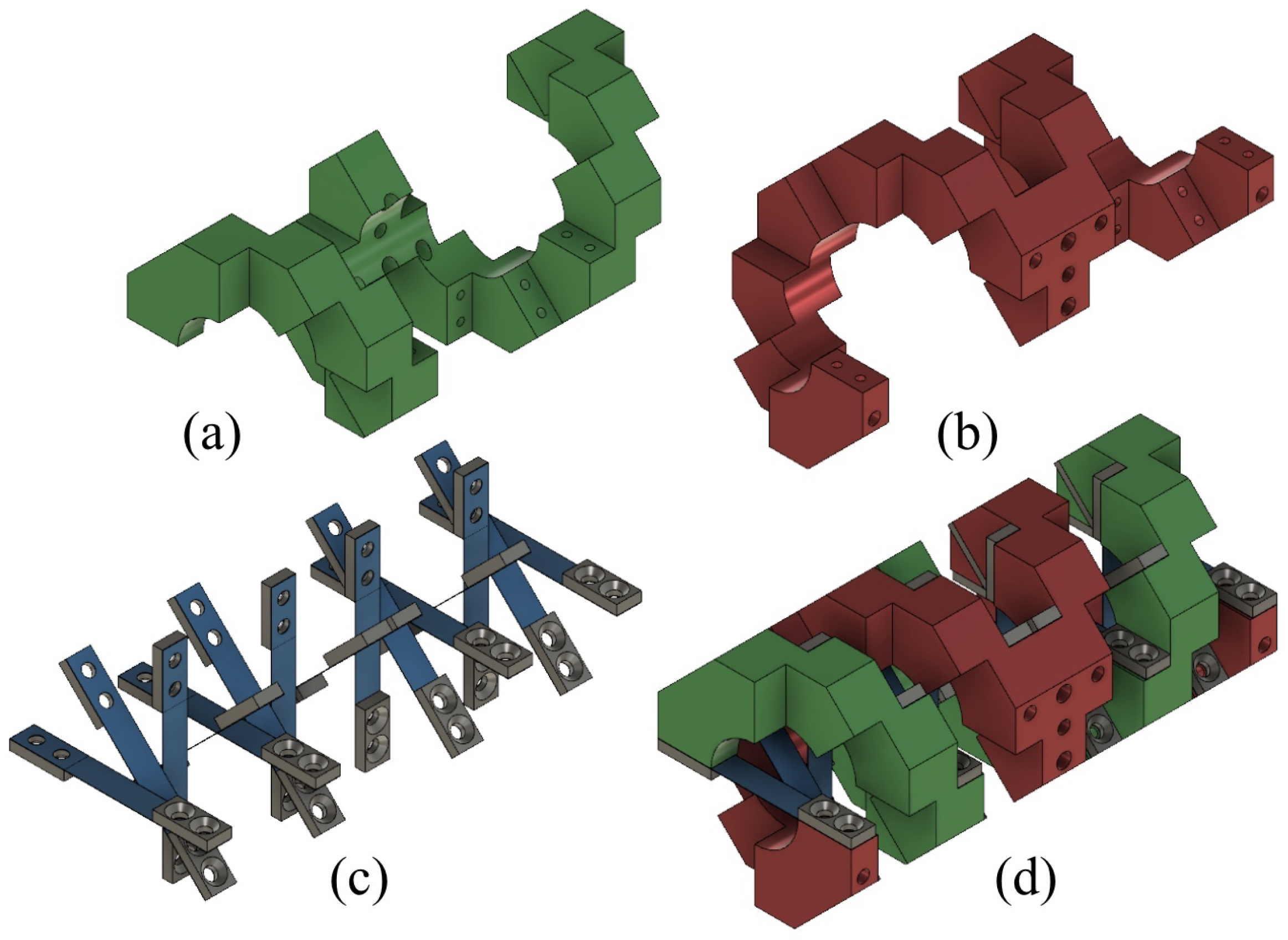Figure 2. Half of a DHCJ: (a) One helical frame with mirror plane. (b) Arrangement of leaf springs.
Figure 2. Half of a DHCJ: (a) One helical frame with mirror plane. (b) Arrangement of leaf springs.Figure 3. Kinematics model of one leaf spring from the DHCJ.
Figure 3. Kinematics model of one leaf spring from the DHCJ.Figure 4. Characteristics testing apparatus.
Figure 4. Characteristics testing apparatus.Figure 5. Comparison of simulation and experimental results.
Figure 5. Comparison of simulation and experimental results.Figure 6. Phase transformation of shape memory alloy (SMA) as a function of temperature.
Figure 6. Phase transformation of shape memory alloy (SMA) as a function of temperature.Figure 7. Dimensions of SMA leaf.
Figure 7. Dimensions of SMA leaf.Figure 8. SMA’s memorized shape.
Figure 8. SMA’s memorized shape.Figure 9. Approximated strain from arc shape of the beam.
Figure 9. Approximated strain from arc shape of the beam.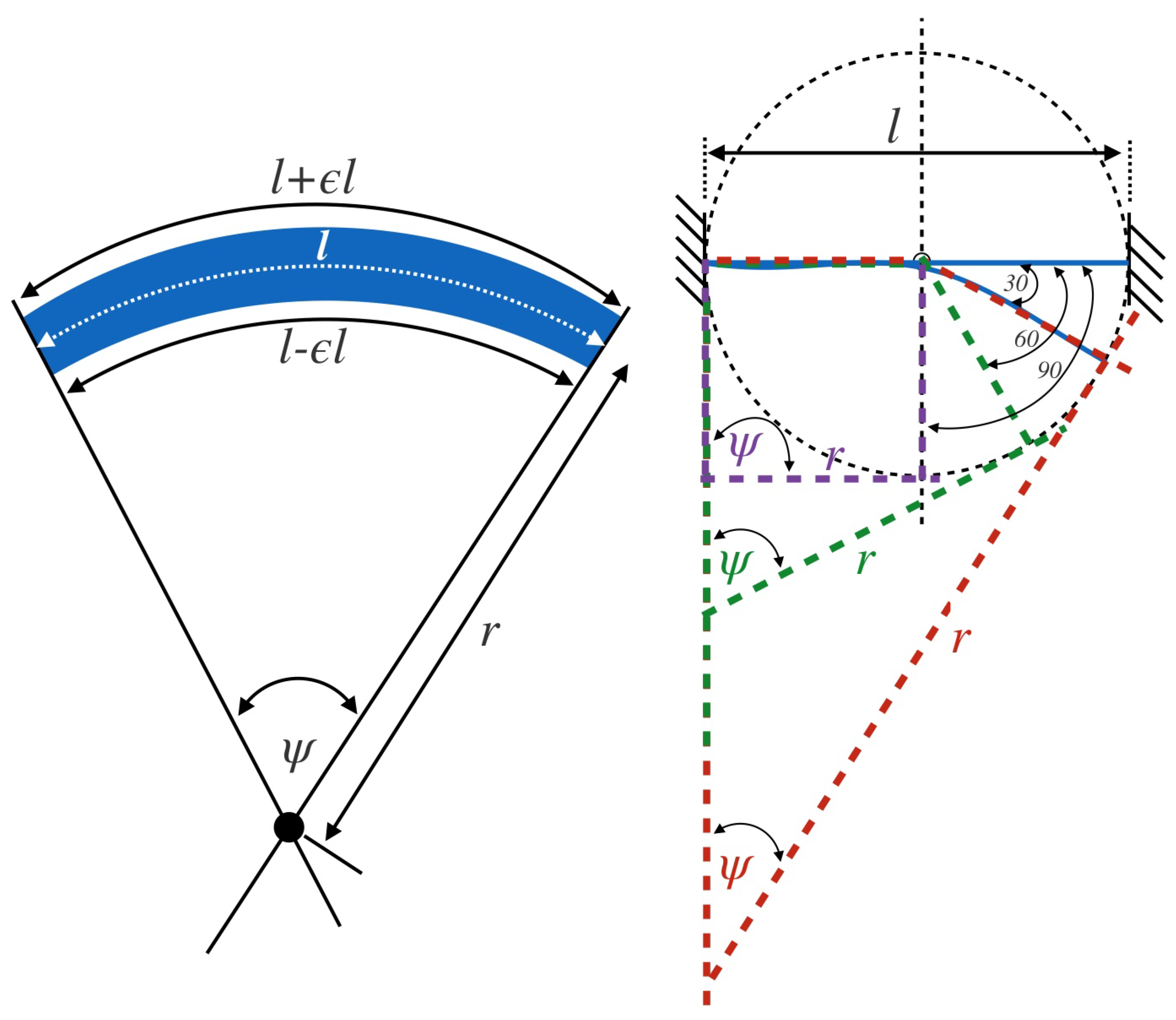Figure 10. Block diagram of the SMA actuator system.
Figure 10. Block diagram of the SMA actuator system.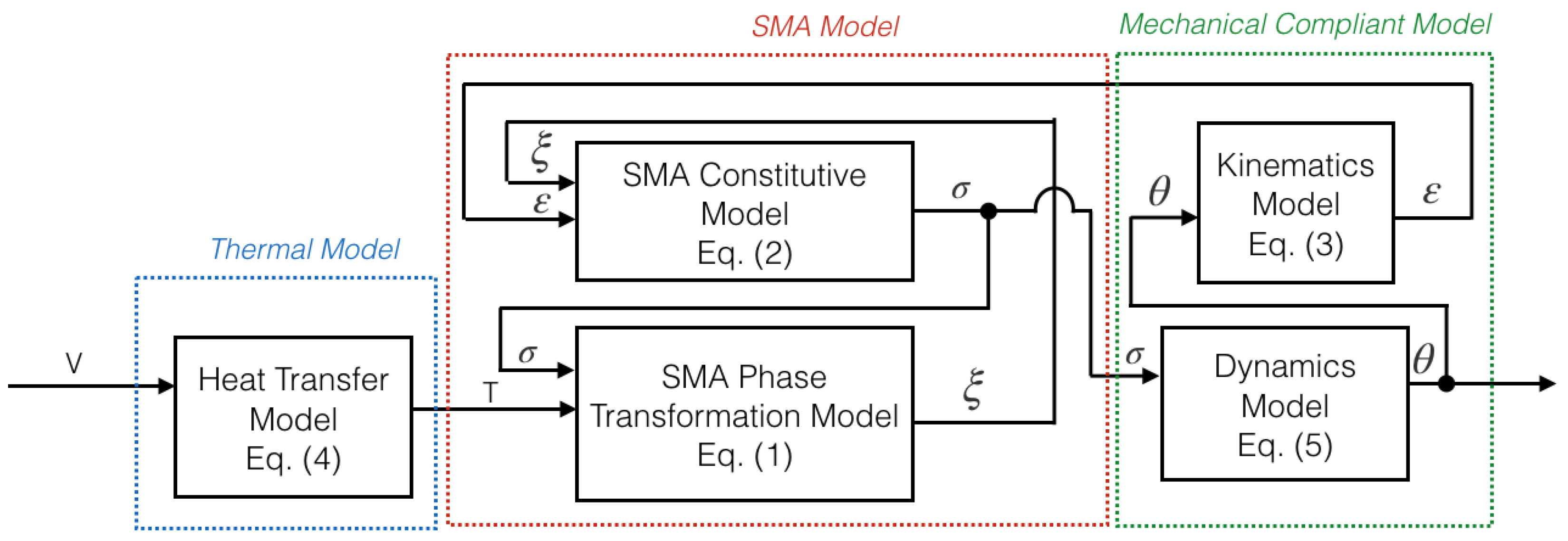Figure 11. Estimated kinematics of DHCJ with an SMA leaf.
Figure 11. Estimated kinematics of DHCJ with an SMA leaf.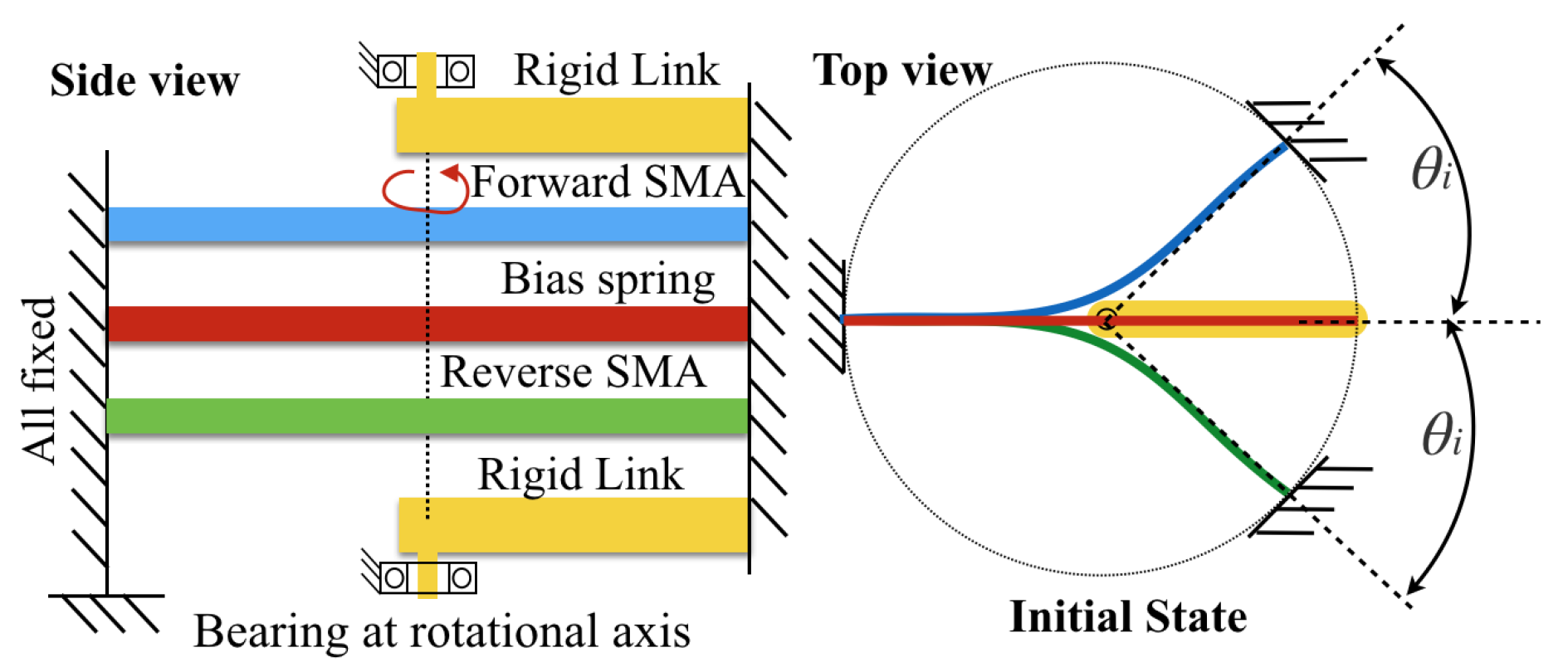Figure 12. Energizing the SMA. (a) Forward drive, (b) reverse drive.
Figure 12. Energizing the SMA. (a) Forward drive, (b) reverse drive.Figure 13. SMA drive circuit.
Figure 13. SMA drive circuit.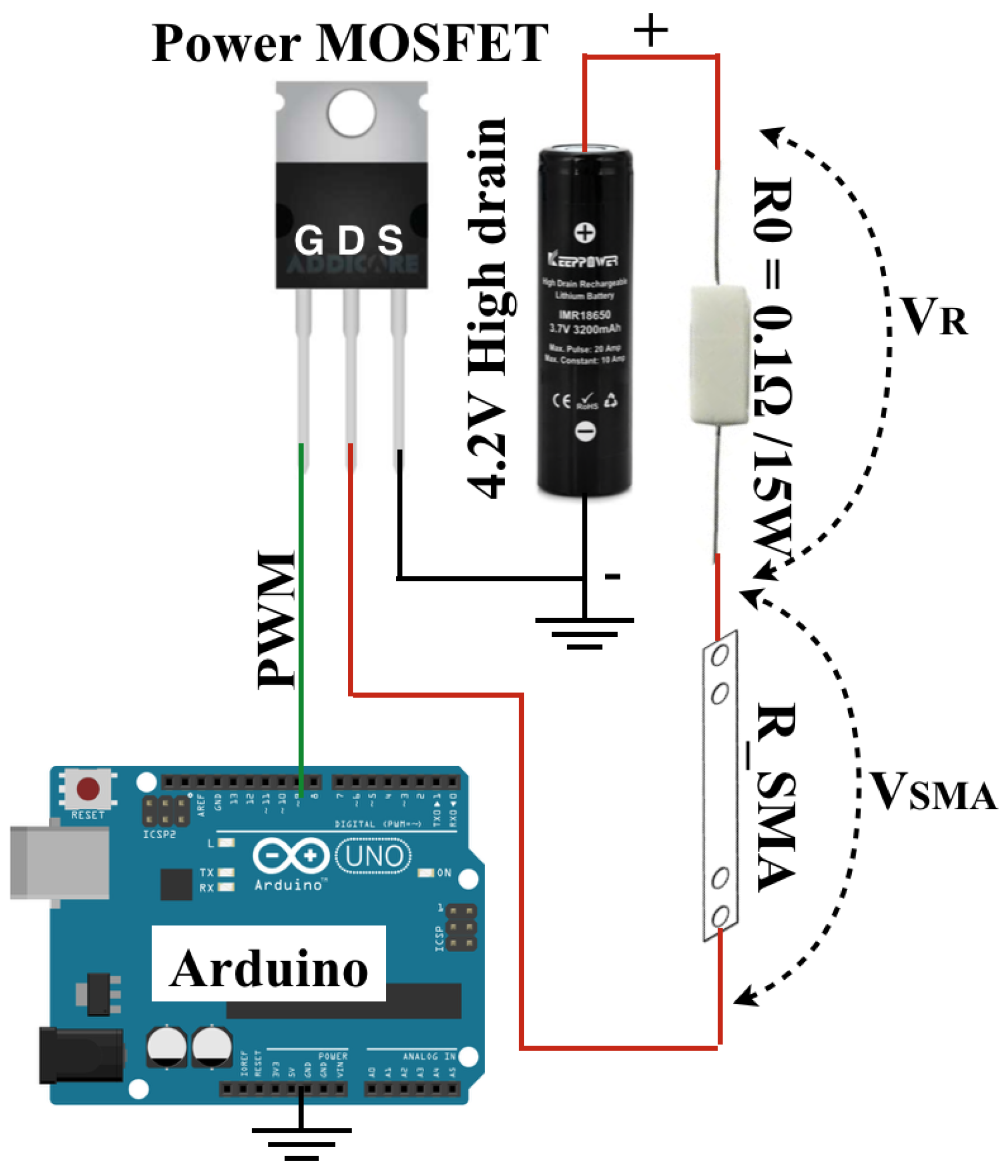Figure 14. Different pre-bent angle of SMA leaf.
Figure 14. Different pre-bent angle of SMA leaf.Figure 15. Deflection angle, temperature with respect to time. (a) “On” period. (b) “Off” period.
Figure 15. Deflection angle, temperature with respect to time. (a) “On” period. (b) “Off” period.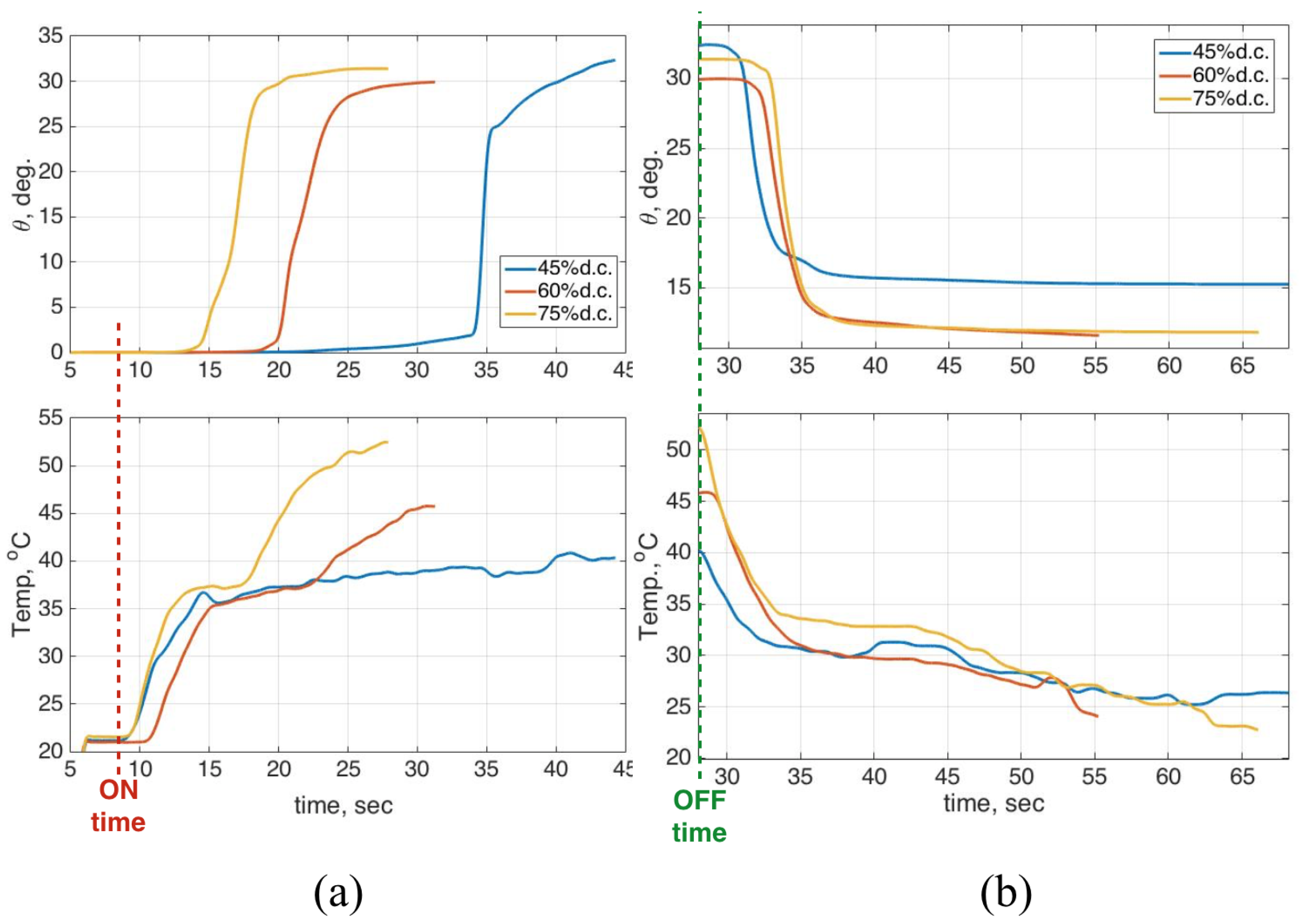Figure 16. CAD model of the SMA actuator. (a) Front view. (b) Perspective view. (c) Arrangement of bias springs and SMA leaves.
Figure 16. CAD model of the SMA actuator. (a) Front view. (b) Perspective view. (c) Arrangement of bias springs and SMA leaves.Figure 17. Rotational stiffness curve of the SMA actuator.
Figure 17. Rotational stiffness curve of the SMA actuator.Figure 18. Apparatus for testing the SMA actuator.
Figure 18. Apparatus for testing the SMA actuator.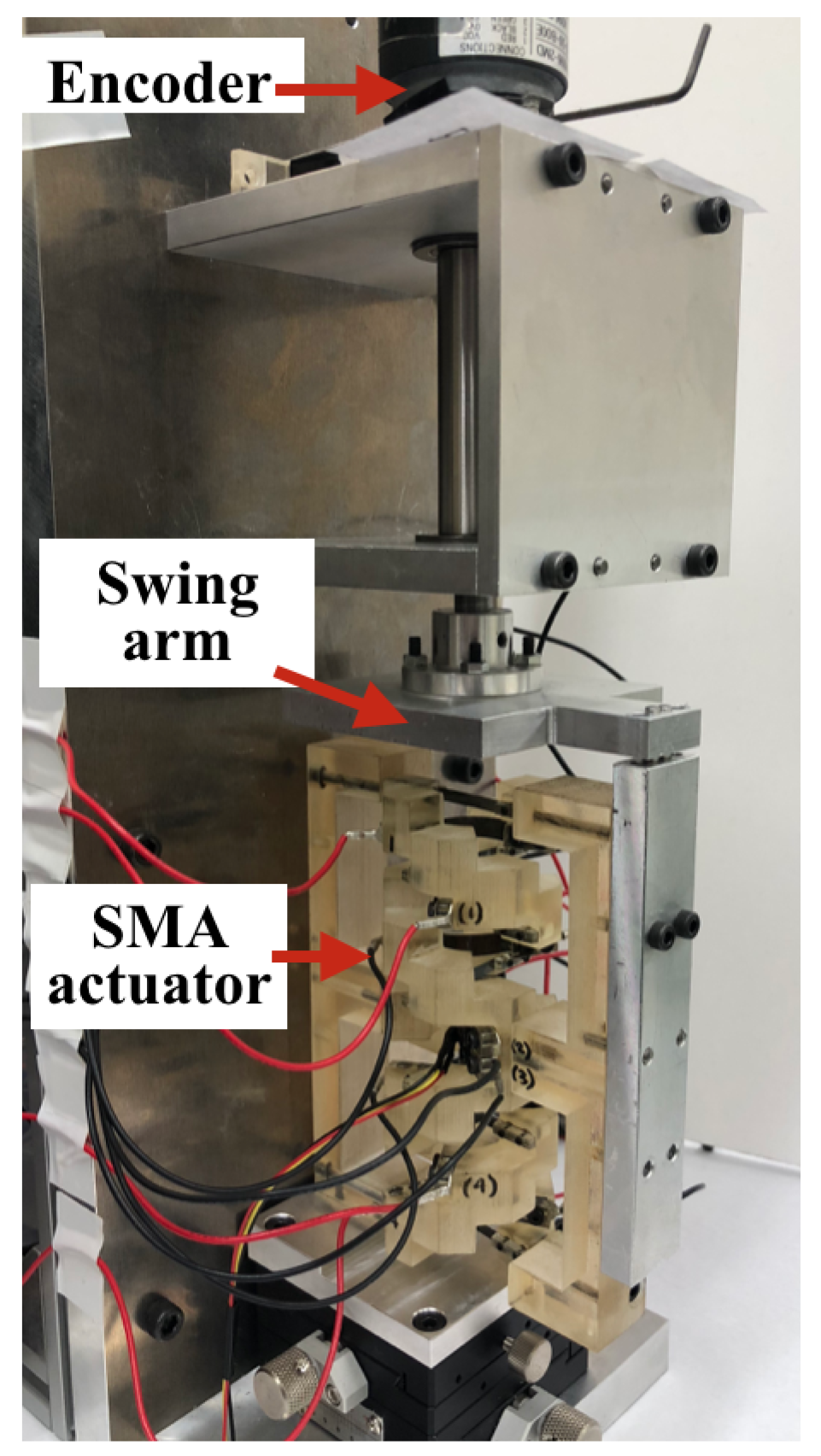Figure 19. Input and output relation.
Figure 19. Input and output relation.Figure 20. Hysteresis loop of angle and temperature.
Figure 20. Hysteresis loop of angle and temperature.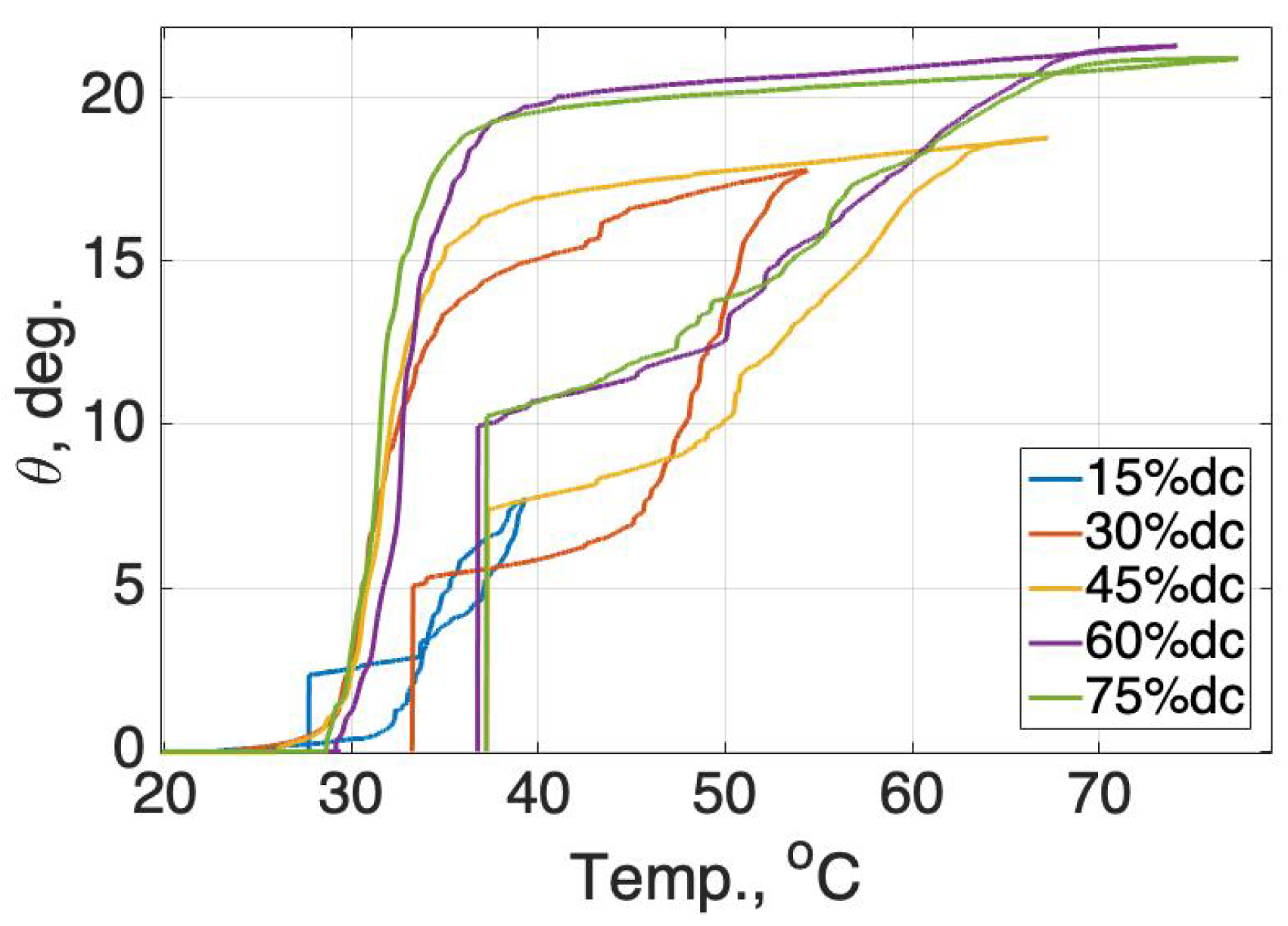Figure 21. Determination of the austenite/martensite temperature.
Figure 21. Determination of the austenite/martensite temperature.Figure 22. Generated torque and temperature with respect to time.
Figure 22. Generated torque and temperature with respect to time.Figure 23. Generated torque with respect to temperature.
Figure 23. Generated torque with respect to temperature.Figure 24. Comparison of torque and deflection angle from experimental and simulation results of the SMA actuator.
Figure 24. Comparison of torque and deflection angle from experimental and simulation results of the SMA actuator.Figure 25. Comparison of a deflection angle with a different input.
Figure 25. Comparison of a deflection angle with a different input.Figure 26. Applying the SMA model as feed-forward control.
Figure 26. Applying the SMA model as feed-forward control.Figure 27. Driving motion of the SMA actuator from top view and side view.
Figure 27. Driving motion of the SMA actuator from top view and side view.Figure 28. Returning motion of the SMA actuator from top view and side view.
Figure 28. Returning motion of the SMA actuator from top view and side view.